• Shuffle
Toggle On
Toggle Off
• Alphabetize
Toggle On
Toggle Off
• Front First
Toggle On
Toggle Off
• Both Sides
Toggle On
Toggle Off
Toggle On
Toggle Off
Front

## Card Range To Study

throughPlay buttonPlay buttonProgress

1/36

Click to flip

Use LEFT and RIGHT arrow keys to navigate between flashcards;

Use UP and DOWN arrow keys to flip the card;

H to show hint;

 There are ___ all the way around a circle. 360 degrees All radii of any circle are ______. Congruent. The diameter is______ times the radius 2 All diameters ___ pass through the center of the circle. must Any angle inscribed inside a circle, with both end points on the diameter, measures ____. 90 degrees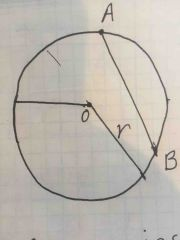Line segment AB is called a ___. ChordLine segment AB is called a ___. Chord Circumference = ___ times the diameter, or 2 ____ times the radius. Pi Area of a circle Pi times the radius squared Area of a circle Pi times the radius squaredLet circle with center O be as shown. Then The measure of angle ADC = 2 times the measure of angle ABC. A ____ is any polygon that has 4 sides. Quadrilateral If a quadrilateral is inscribed in a circle, then ___. Opposite corners' angles are supplementary. All the angles in a quadrilateral add up to ___. 360 degrees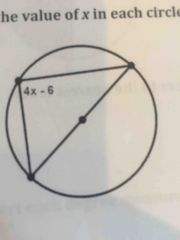x = ___ 24 degrees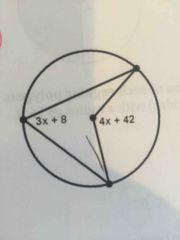x = ___ 13 degrees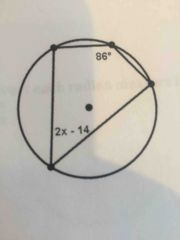x = ___ 54 degrees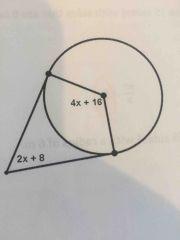x = ___ 26 degrees Any time the thing you're solving for is on the bottom of one side of the equation, ___. it switches places with the whole other side of the equation.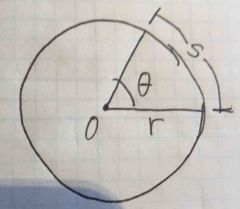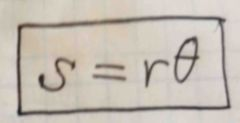Area of a regular polygon with n sides and radius r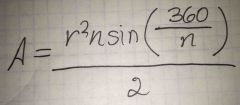Back (Definition) Perimeter of a regular polygon with n sides and radius r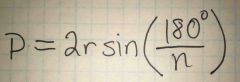Back (Definition)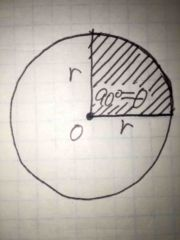Front (Term)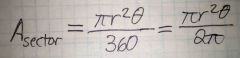Back (Definition) 45 degrees is ___ of 180 degrees 1/4 45 degrees is ___ radians. pi/4 Pi/4 radians is ___ degrees. 45 150 degrees is ___ of 180 degrees. 5/6 150 degrees is ___ radians. 5pi/6 5pi/6 radians is ___ degrees. 150 75 degrees is ___ of 180 degrees. 5/12 75 degrees is ___ radians. 5pi/12 5pi/12 radians is ___ degrees. 75 Distance formula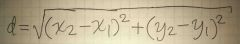Midpoint formula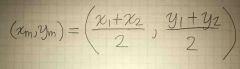Back (Definition) Formula for the slope of a line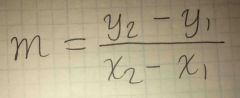Back (Definition) Slope of the perpendicular line Opposite reciprocal of the slope of the original line